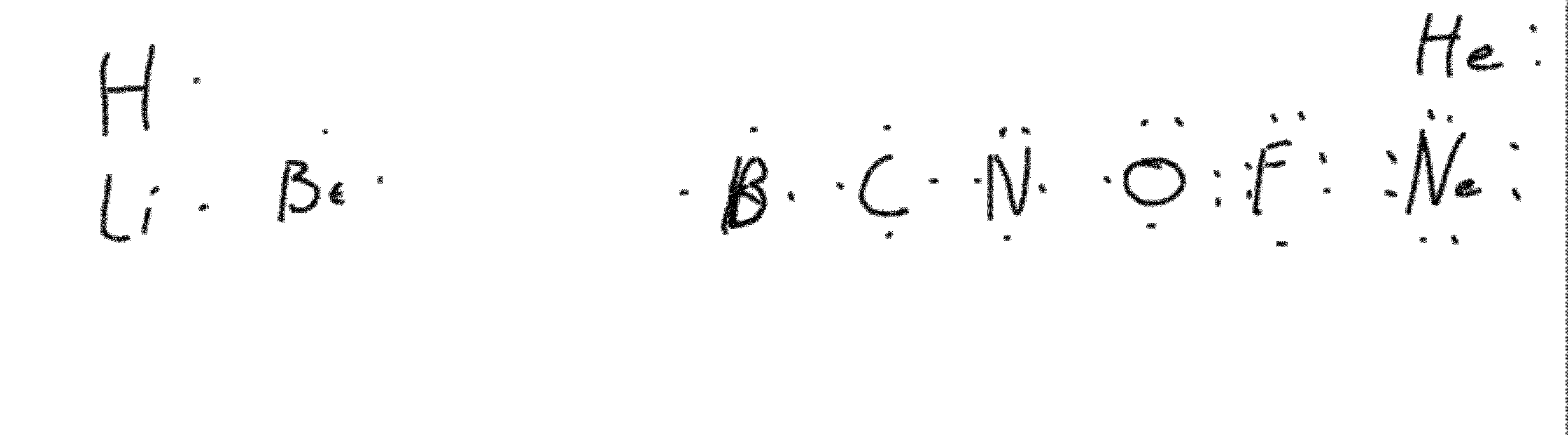## Blog Entries

#### Intro Kinetics

Introduction to Kinetics.
Some reactions are slow (oxidation of iron in air to make rust), some very fast (oxidation of C4 explosives).
Oxidation of sugar releases more energy than oxidation of the same mass of TNT…yet, burning sugar does not release energy fast enough to explode.

## Here is a link to a practice worksheet on kinetics.Pogil Answers.

Here is a link to the answer keys for the Pogil activities. First one is ready now.
First Pogil Answers. Note that the answers should be good…but, if you have different answers, don't be concerned. I make mistakes.
Here is the second one.

Also, here are some reaction mechanism problems:

Below is an overall reaction and a proposed reaction mechanism:

Overall:
2NO2Cl(g) 2NO2(g) + Cl2(g)

1. NO2Cl NO2 + Cl (slow)
2. NO2Cl + Cl NO2 + Cl2 (fast)

Assuming the reaction mechanism above is correct
1. What is the rate determining step?
2. What should be the rate law for the overall reaction?
3. What reaction intermediate is present?

The rate determining (limiting) step is the first one. That is, the slow one.
The rate law should be Rate=k[NO2Cl]
The reaction intermediate is Cl a chlorine atom.

#### Short Problems

I added a second problem to practice.MathJax Font